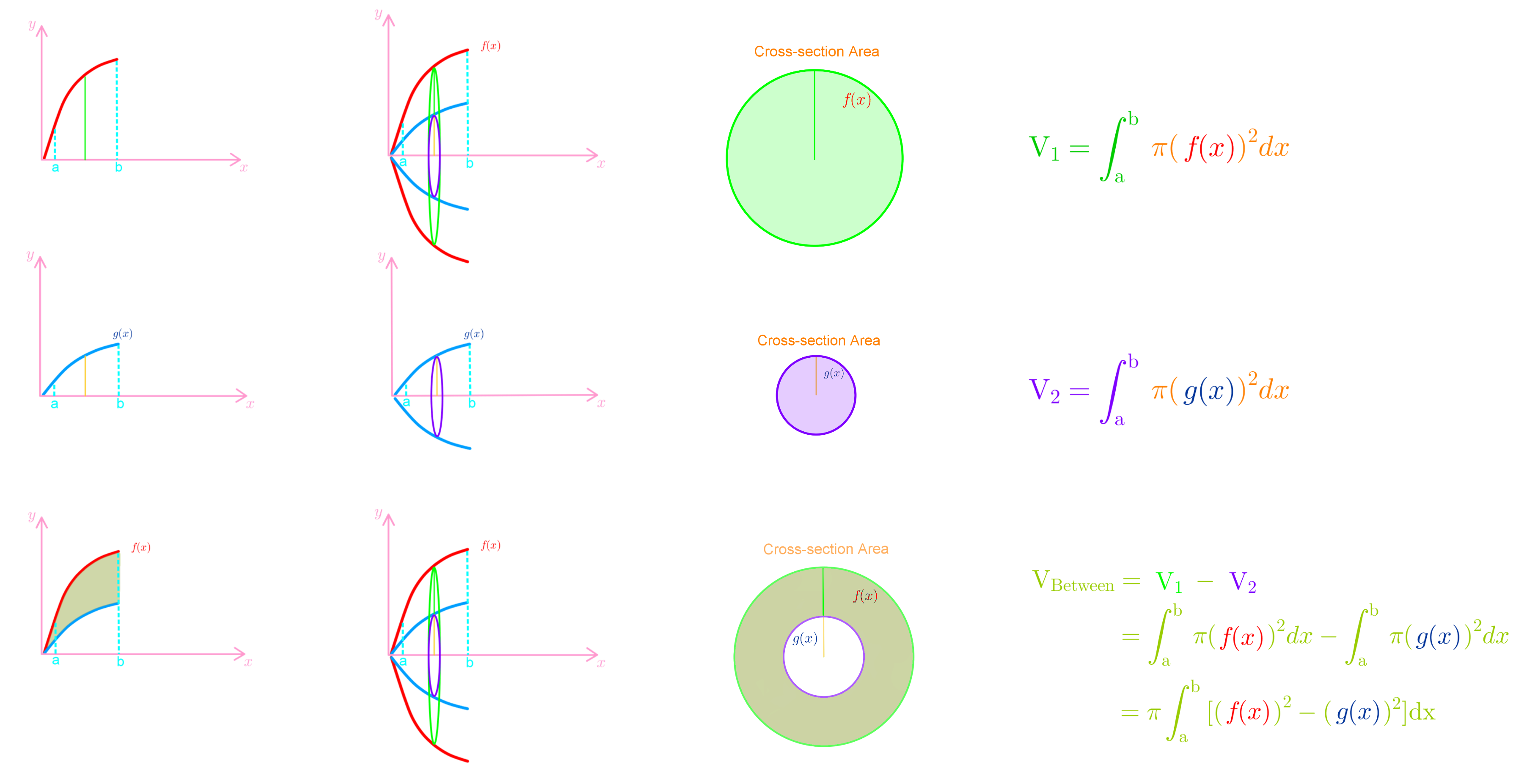Volumes of solids of revolution - Disc method

Volumes of solids of revolution - Disc method

In this lesson, we learn that rotating the function around the x-axis will give us a solid. This is known as the disc method. You notice that the cross sections of the solid are always circles. This is important because we know the area of the circle, and integrating this will give us the volume. We also learn that the radius of the circle is simply the function itself. In more advanced questions, we see that we can use the region between two curves and rotate it around the x-axis. However, the cross section is the region between the two circles. Hence, the radius will be the outer radius minus the inner radius. In this section, you will also deal with questions which involve rotating functions around the y-axis.

Lessons• Introduction

• 1.
The region bounded by the graphs of $y=e^x$, $y=0$,$x=0$, and $x=1$ is revolved about the x-axis. Find the volume of the resulting solid.

• 2.
Find the volume of the solid obtained by rotating the region bounded by $x=3\sqrt{(y-1)}$, $x=0$, and $y=2$ about the y-axis.

• 3.
Find the volume of the solid obtained by rotating the region bounded by $y=\sqrt{(x+1)}$, and $y=\frac{1+x}{2}$ about the x-axis.

• 4.
Find the volume of the solid obtained by rotating the positive region bounded by $y=x^3$, $y=x$
a)
about $y=3$

b)
about $x=-2$

• 5.
Find the volume of the solid obtained by rotating the region bounded by $y=3-cosx$, and $y=4$ about $y=1$.